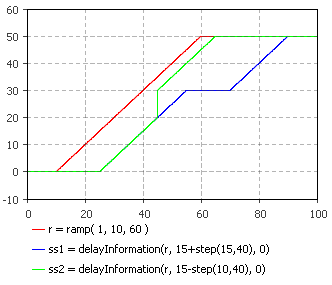AnyLogic

# delayInformation

delayInformation is the system dynamics function that returns the value of the input delayed by the delay time.

The initial value is the value of the variable on the left-hand side of the equation at the start of the simulation. The delay time can be a variable. If delay time is decreasing some values of the input will be discarded and replaced by more recent inputs. If delay time is increasing existing values will be held.

The plot below illustrates how the function works:This function can be called in formulas of system dynamics variables and has the following notation:

• delayInformation(input, delayTime, initialValue)

input — can be a flow variable, or a numeric expression of any complexity.

delayTime — can be either a constant or a numeric expression (e.g. a function call, or a numeric parameter).

initialValue — initial value.

#### Units

input — unit

delayTime — time

initialValue — unit

delayInformation() — unit

The output units are the same as the input ones.